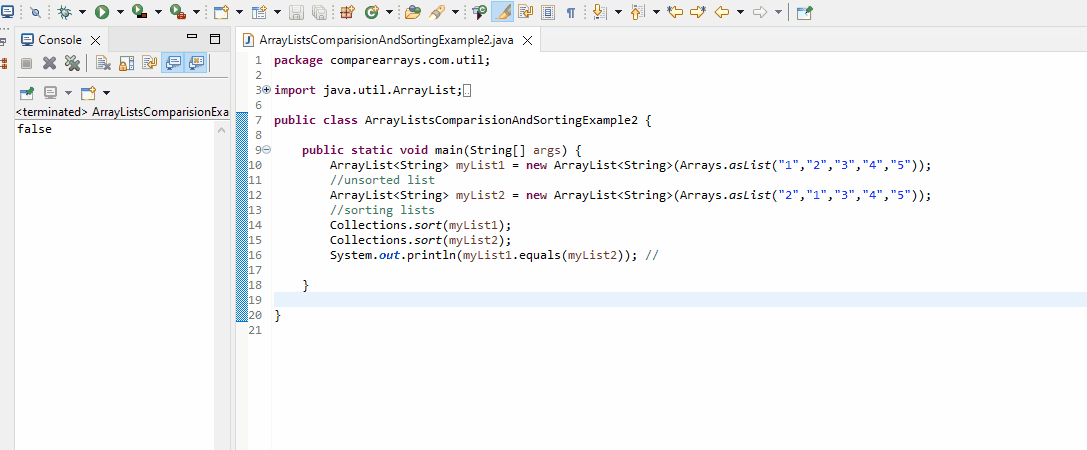# Compare ArrayLists in Java

Sarwan Soomro Jan 03, 2023 Apr 01, 2022 Java Java Method Java ArrayList

Java provides straightforward methods for comparing string classes and array lists and whatnot. So, we’ll keep it simple and clean for you.

In this tutorial, first, we will compare two array lists using a comparison method in Java. We also apply the same method to Java strings before applying it to array lists.

Finally, we demonstrate how you can sort an unordered array list before comparison.

## Compare Array Lists in Java

Java has an inbuilt method to compare objects, size, and elements within arrays lists. Although there are many methods we can use to get the same output, we will use the following method since it is the most straightforward.

### the `.equals()` Function in Java

This function in Java compares the user-specified object to this list.

Syntax Structure:

``````ArrayList1.equals(ArrayList2)
``````

Parameter: An object only; in this case, `ArrayList2` is an object being passed as a single parameter.

This method will return Boolean’s `true` or `false` based on the following.

1. If the user-specified object is also a list.
2. Both lists have the same size.
3. All related pairs of elements in the two lists are also equivalent.

To understand this method more objectively, check out the following program we have created using strings.

Later, we will use the same logic on the array lists.

### Use the `.equals()` Method to Compare Strings in Java

``````package comparearrays.com.util;

public class FirstStringDemoEqualsMethd {

public static void main(String[] args) {
String one = "USA";
String two = "USA";
String three = "Germany";

// comparing the values of string one and string two
if (one.equals(two) == true) {
System.out.println("String one: "+ one);
System.out.println("String one: "+ two);
System.out.println("Both are equal");
}
else {
System.out.println("Both are not equal");
}

// compares string one's values against string three's (since both are the same, true)
// false (one and three are not the same, false)
System.out.println("String one and String three are not the same: so, " +one.equals(three));

}

}
``````

Output:

``````String one: USA
String one: USA
Both are equal
String one and String three are not the same: so, false
``````

## Compare Two Array Lists in Java

We used `.equals()` to compare string values in the example above to show that the method will work the same when using it on `ArrayList` in Java. How so?

Check the following Java syntax:

``````ArrayList<String> myList1 = new ArrayList<String>();
ArrayList<String> myList2 = new ArrayList<String>();
``````

The Array Lists use Java’s `String` class here. Likewise, we compared strings in the previous example.

And now, we will use the same method on `ArrayList<String>`.

Got it all clear? Let’s do it.

Java Code:

``````package comparearrays.com.util;

import java.util.ArrayList;

//main class
public class ArrayListComparisionExample1 {
// main function
public static void main(String[] args) {

// list 1
ArrayList<String> myList1 = new ArrayList<String>();
// values
// list 2
ArrayList<String> myList2 = new ArrayList<String>();
// values
// print list 1
System.out.println("Values from list 1: " + myList1);
// print list 2
System.out.println("Values from list 2: " + myList2);

// apply .equals() method for comparision
if (myList1.equals(myList2) != false) {
System.out.println("Both Array Lists are equal!");
} else {
System.out.println("Both Array Lists are not equal!");
}

// add new values to list to determine if condition works
// print updated list
System.out.println("Updated valued from list 1: " + myList1);

// compare again

if (myList1.equals(myList2) != false) {
System.out.println("Both Array Lists are equal!");
} else {
System.out.println("Both Array Lists are not equal!");
}

}

}
``````

Output:

``````Values from list 1: [Apple, Orange, Grapes, Water Melon]
Values from list 2: [Apple, Orange, Grapes, Water Melon]
Both Array Lists are equal!
Updated valued from list 1: [Apple, Orange, Grapes, Water Melon, Pine Apple]
Both Array Lists are not equal!
``````

## Sort Array Lists Before Comparison in Java

What if your array lists have the same size and elements, but the values are not sorted?

``````ArrayList<String> myList1 = new ArrayList<String>(Arrays.asList("1","2","3","4","5"));
ArrayList<String> myList2 = new ArrayList<String>(Arrays.asList("2","1","3","4","5"));
``````
Note
The simple solution is to use `import java.util.Collections;`. It will do the rest for you. Here is how.
``````package comparearrays.com.util;

import java.util.ArrayList;
import java.util.Arrays;
import java.util.Collections;

public class ArrayListsComparisionAndSortingExample2 {

public static void main(String[] args) {
ArrayList<String> myList1 = new ArrayList<String>(Arrays.asList("1","2","3","4","5"));
//unsorted list
ArrayList<String> myList2 = new ArrayList<String>(Arrays.asList("2","1","3","4","5"));
//sorting lists
Collections.sort(myList1);
Collections.sort(myList2);
System.out.println(myList1.equals(myList2)); //

}

}
``````

Output:

``````true
``````

If we do not use `Collections.sort(myList2);`, the output will be `false`.

You can apply the same `sort()` method to unordered values but have the same objects and elements. Then, you can use `.equals()`.

Live demo:Sarwan Soomro is a freelance software engineer and an expert technical writer who loves writing and coding. He has 5 years of web development and 3 years of professional writing experience, and an MSs in computer science. In addition, he has numerous professional qualifications in the cloud, database, desktop, and online technologies. And has developed multi-technology programming guides for beginners and published many tech articles.

## Related Article - Java Method

• Method Chaining in Java
• Optional ifPresent() in Java
• Override and Overload Static Methods in Java
• Use of the flush() Method in Java Streams
• Use the wait() and notify() Methods in Java
• Custom Helper Method in Java
• ## Related Article - Java ArrayList

• Sort Objects in ArrayList by Date in Java
• Find Unique Values in Java ArrayList
• Vector and ArrayList in Java
• Differences Between List and Arraylist in Java
• Merge Sort Using ArrayList in Java